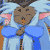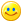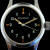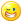## Welcome to the Second Life Forums Archive

These forums are CLOSED. Please visit the new forums HERE

# Float to Scientific Notation (safe transport for floats through strings)

Strife Onizuka
MoonchildJoin date: 3 Mar 2004
Posts: 5,887
11-22-2004 07:14
A script for passing floats through strings without loosing precision.
wrote this ages ago, it isn't very fast but it is accurate.

Works flawlessly and LSL can parse directly to floats again without any special codejust use a (float) typecast.

Changes:
Rewrote the code almost from scratch, imported much of the knowledge learned from Float2Hex and float-union-integer (shares some code with FUI). Optimized the code, added comments, and descriptive variable names. Fixed a crashing bug and added precision to the math (won't effect the output). Relaxed some of the string method to be faster. Basically, everything has changed. It should also be *faster*. Cleaner logic.

CODE
`string Float2Sci(float input){	if(input == 0.0)//handles negitive zero		return llDeleteSubString((string)input, -5, -1);//trim off the trailing zero's, don't need them.		float frac = llFabs(input);//we put the negitive back at the end.	string mantissa = (string)frac;//this may be a string of about 47 characters long.	integer exponent = -6;//default exponent for optical method	if(frac < 16.0)//optical might fail		if(frac != (float)mantissa)//optical did fail			jump in;	//optical will work, so all we need do is remove the decimal point and jump to optical.	mantissa = llDeleteSubString(mantissa, -7, -7);	jump optical;	@in;	//Ugly Math version; ugly in the sence that it is slow and not as elegant as working with it as a string.	//A) calculate the exponent via approximation of C log2()	//B) use cludge to avert fatal error in approximation of log2 result (only a problem with values >= 0x1.FFFFF8p127)	//       the exponent is sometimes reported as 128, which will bork float math, so we subtract the test for 128.	//       max_float btw is 0x1.FFFFFEp127, so we are only talking a very small number of numbers.	//C) normalize the float with questionable exponent	//D) calculate rounding error left from log2 approimation and add to normalization value.	//       the '|' acts like a '+' in this instance but saves us one byte.	integer position = (24 | (3 <= frac)) - (integer)( //D			frac /= (float)("0x1p"+(string)( //C			exponent = (exponent - (( //B				exponent = llFloor(llLog(frac) / 0.69314718055994530941723212145818) //A			) == 128))			))			);		//this pushes the float into the interger buffer exactly.	//since the shift is within integer range, we don't need to make a float.	integer int = (integer)(frac * (1 << position));	integer target = (integer)(frac = 0.0);//since the float is in the integer buffer, we need to clear the float buffer.		//we don't use a traditional while loop, and instead opt for a do-while, because it's faster	//since we may have to do about 128 iteration, this savings is important,	//the exponent needs one final adjustment because of the shift, we do it here to save memory & it's fasfter.		//The two loops try to make exponent == position by shifting and multiplying.	//when they are equal, then this should be true ((int * llPow(10, exponent)) == llFabs(input))	//That is of course assuming that the llPow(10, exponenet) result has enough percision.		//We recycle position for these loops as a temporary buffer. This is so we can save a few operations.	//If we didn't, then we could actualy optimize the variable out of the code; though it would be slower.	if(target > (exponent -= position))	{//apply the rest of the bit shift if |input| < 1		do		{			if(int < 0x19999999)//(0x80000000 / 5)			{//won't overflow, multiply in 5				int = int * 5 + (position = (integer)(frac *= 5.0));				frac -= (float)position;				target = ~-target;			}			else			{//overflow predicted, devide by 2				frac = (frac + (int & 1))/2;				int = int >> 1;				exponent = -~exponent;			}		}while(target ^ exponent);	}	else if(target ^ exponent)//target < exponent	{//apply the rest of the bit shift if |input| > 1		do		{			if(int < 0x40000000) //(0x80000000 / 2)			{//won't overflow, multiply in 2				int = (int << 1) + (position = (integer)(frac *= 2.0));				frac -= (float)position;				exponent = ~-exponent;			}			else			{//overflow predicted, devide by 5				frac = (frac + int%5) / 5.0;				int /= 5;				target = -~target;			}		}while(target ^ exponent);	}	//int is now properly calculated, it holds enough data to accurately describe the input in conjunction with exponent.	//we feed this through optical to clean up the answer.	mantissa = (string)int;	@optical;	//it's not an issue that we may be jumping over the initialization of some of the variables,	//we initialize everything we use here.		//to accurately describe a float you only need 9 decimal places; so we throw the extra's away	if(9 < (target = position = llStringLength(mantissa)))		position = 9;	//chop off the tailing zero's; we don't need them.	do; while(llGetSubString(mantissa, position, position) == "0" && (position = ~-position));//faster then a while loop		//we do a bad thing, we recycle 'target' here, position is one less then target,	//"target + ~position" is the same as "target - (position + 1)" saves 6 bytes.	//this block of code actualy does the cutting.	if(target + ~position) mantissa = llGetSubString(mantissa, 0, position);		//insert the decimal point (not strictly needed). We add the extra zero for asthetics.	//by adding in the decimal point, it simplifies some of the code.	mantissa = llInsertString(mantissa, 1, llGetSubString(".0", 0, !position));	//adjust exponent from having added the decimal place	if((exponent += ~-target))		mantissa += "e" + (string)exponent;	//return with the correct sign.    if(input < 0)        return "-" + mantissa;    return mantissa;}`

Old Version:
CODE
`float LOG2 = 0.30102999566398119521373889472449;string FloatToSci(float Feed) {     integer c;    integer d;    integer e;        float AFeed = llFabs(Feed);    integer Exp;    string Mant = (string)AFeed;        if(AFeed<16.0) //unsure about optical    {        if(AFeed!=(float)Mant) //optical fails        {            //55 bits used.            //d is the bitshift            //AFeed is the             integer f;            float tff;            c=(integer)((AFeed / llPow(2,d = llFloor(llLog10(AFeed) / LOG2))) * llPow(2,24));            d -= 24;                        //time to apply the rest of the bit shift AFeed<1            while(e>d)            {                //overflow test                if(c >= 0x19999999) //(0x80000000 / 5)                {//overflow predicted, devide by 2                    tff = (tff + c%2)/2;                    c/=2;                    ++d;                }                else                {//won't overflow, multiply in 5                    c = c*5 + (f = (integer)(tff*=5.0));                    tff -= (float)f;                    --e;                }            }            //time to apply the rest of the bit shift AFeed>1            while(e<d)            {                if(c >= 0x40000000) //(0x80000000 / 2)                {//overflow predicted, devide by 5                    tff = (tff + c%5) / 5.0;                    c /= 5;                    ++e;                }                else                {//won't overflow, multiply in 2                    c= c * 2 + (f = (integer)(tff *= 2.0));                    tff -= (float)f;                    --d;                }            }            Mant = (string)c;            Exp = d;            //use this to speed things up, but output will be ugly and possibly wasteful.//            jump quick;            d = llStringLength(Mant);            jump go;        }    }    d = llStringLength(Mant);    if((c = llSubStringIndex(Mant,"."))+1)    {//remove the decimal, this makes everything else easier.        Exp -= (d -= 1) - c;        Mant = llDeleteSubString(Mant,c,c);    }    @go;    if(d>9 || llGetSubString(Mant,-1,-1)=="0")    {        if((e = d)>9)            c = 9;        else            c = d - 2;//already checked the last digit.        while(llGetSubString(Mant,c,c)=="0" && c)                --c;        Exp += e - (d = (c + 1)) + (c!=-1)*c;        Mant = llInsertString(llDeleteSubString(Mant, c+1, e), 1, llGetSubString("0.0", d>0, 1 + (d<2)));    }    else    {        if(d)            Exp += d - 1;        Mant = llInsertString(Mant, 1, llGetSubString("0.0", d>0, 1 + (d<2)));    }    @quick;    if(Exp)    {        if(Exp>0)            Mant += "E+" + (string)Exp;        else            Mant += "E" + (string)Exp;    }    if(Feed<0)        return "-" + Mant;    return Mant;}`
_____________________
Truth is a river that is always splitting up into arms that reunite. Islanded between the arms, the inhabitants argue for a lifetime as to which is the main river.
- Cyril Connolly

Without the political will to find common ground, the continual friction of tactic and counter tactic, only creates suspicion and hatred and vengeance, and perpetuates the cycle of violence.
- James Nachtwey
Francis Chung
This sentence no verb.Join date: 22 Sep 2003
Posts: 918
12-01-2004 19:45
Hi StrifeThis is a pretty neat bit of code. Very useful, because there's no simple loss-less way of passing a floating value from 1 script to another.

Do you think that you could add comments/rename variables? I have trouble understanding what certain portions of code are supposed to do. Perhaps a less-optimized version of this script would be handy.
_____________________
--
~If you lived here, you would be home by now~
Strife Onizuka
MoonchildJoin date: 3 Mar 2004
Posts: 5,887
12-10-2004 03:43
I suppose. I've been meaning to rewrite parts of it anyway. But in the mean time i'll tell discribe what is being done.

CODE
`    if(llFabs(tf)>=16.0)     {         string ttt=(string)llFabs(tf);         integer tfi;         integer tff;         ti=6;         for(tff=llStringLength(ttt) - 1;1+tfi=llSubStringIndex(".0",llGetSubString(ttt,tff,tff));--tff)             ti-=tfi;         if(ti>0)             ti=0;         else if(tff>8)         {             ti+=8 - tff;             tff=8;         }         taa=llDeleteSubString("- ",(tf<0),-1)+llGetSubString(ttt,0,tff);     }     else `

This section of code just converts the float to a string then cuts it so it only uses 8 characters, 8 digits is the number needed to accuratly represent a float. any number greater then 16 doesn't need special handling.
first we remove the sign so we don't ahve to worry about it. We will add it back later.
conver the float to a string
then we strip off zero's from the string and the decimal place if we can.
Then we count the number of characters left, if they exceed 8 characters we cut it to 8 characters.
the number of characters chopped excluding any that were chopped before the decimal is the exponant.

CODE
`    else     {         //55 bits used.         //whats the bit shift?         ti = -llFloor(llLog10(llFabs(tf)) / 0.30102999566398119521373889472449);         tf*=llPow(2,ti);              //time to tweak the bit shift some...         integer ttt=24;         float tff=tf*llPow(2,ttt);         while(tff+1.0==tff || 0==(1&(integer)tff))             tff=tf*llPow(2,--ttt);         integer tfi=(integer)tff;         ti+=ttt;         ttt=0;         tff=0;         //time to apply the bit shift |tf|<1         while(ttt<ti)         {             if((5*tfi)/5==tfi)             {                 tfi=tfi*5+(integer)(tff*=5.0);                 tff-=(float)((integer)tff);                 ++ttt;             }             else             {                 tff=(tff + tfi%2)/2;                 tfi=tfi/2;                 --ti;             }         }         //time to apply the bit shift |tf|>1         while(ttt>ti)         {   //((1073741824&tfi)*2  == (tfi&-2147483648)) //             if((0x40000000&tfi)<<1 == (tfi& 0x80000000))               {                 tfi=tfi*2+(integer)(tff*=2.0);                 tff-=(float)((integer)tff);                 ++ti;             }             else             {                 tff=(tff + tfi%5)/5.0;                 tfi=tfi/5;                 --ttt;             }         }         taa=(string)tfi;     } `

This section of code deals will all floats less then 16 these *require* special handling. The problem with multiplying the number by 10 to push it into range is that the least significant bits can become corrupt.
The solution is to shift the number by a power of 2 so it is an integer. Then we multiply in the 5's (or devide out the fives depending on which way the shift was) We use a float and integer to reperest the number, giving us 55 bits to work with; more then enough. We do special checking to make sure the integer doesn't overflow and if it is we instead of multiplying (or deviding) in the 5 we devide out a 2 (or multiple out). Any float part left over from doing this on the integer is transfered over to the float.
Finaly when the number of 2's equals the number of 5's we discard the float and convert the integer to a string.

CODE
`    if(-1==llSubStringIndex(taa,".")) taa+=".0";     if(ti) taa+="E"+llDeleteSubString("+ ",(ti<=0),-1)+(string)(-ti);     return taa; `

we add a decimal point if there isn't on (not required by LSL)
then add the exponential value. (the plus sign isn't required)
your probably wondering why it's negative. Well i wrote the less then 16 handler first. And when i wrote it i accidentily made it negative. So instead of going back and changing the very scary looking code i left it.

Since i have some time i think i'll rework this so it's less scary. And hopefully faster. I have a few ideas how to make it less so.
_____________________
Truth is a river that is always splitting up into arms that reunite. Islanded between the arms, the inhabitants argue for a lifetime as to which is the main river.
- Cyril Connolly

Without the political will to find common ground, the continual friction of tactic and counter tactic, only creates suspicion and hatred and vengeance, and perpetuates the cycle of violence.
- James Nachtwey
Strife Onizuka
MoonchildJoin date: 3 Mar 2004
Posts: 5,887
01-27-2005 14:09
I've rewriten parts of both optical & mathimatical methods. Everything now goes through optical as this does a nice job of cleaning up after mathamatical. Also I rewrote much of the optical method to allow for this.

The code doesn't scare me so much now.

About the code. Lettered variables are temp variables. They get recycled after thier values are no longer needed.

The major change in the mathimatical method was that it no longer use keeps track of the sign, it's added back on at the very end.

Optical was changed to be able to handle numbers without decimal points. This lets it parse the results from mathimatical. Optical was also changed to properly parse smaller numbers.

Runs about as fast as it did before.

EDIT:
Just changed where the decimal place is placed when it is not present. Should make nicer looking floats (numbers that where forced through mathimatical like "1.24415647" come out as "1.24415647" instead of "124415647E-8"How to make this script run faster:
Uncomment the jump in the nested if statements
comment out the code that inserts the decimal point. (6 lines of code below @end); this actualy isn't nessesary for LSL string -> float convertion.
and replace
if(Exp) Mant+=llGetSubString("E+",0,(Exp>0)) + (string)Exp;
with
if(Exp) Mant+="E" + (string)Exp;
_____________________
Truth is a river that is always splitting up into arms that reunite. Islanded between the arms, the inhabitants argue for a lifetime as to which is the main river.
- Cyril Connolly

Without the political will to find common ground, the continual friction of tactic and counter tactic, only creates suspicion and hatred and vengeance, and perpetuates the cycle of violence.
- James Nachtwey
Strife Onizuka
MoonchildJoin date: 3 Mar 2004
Posts: 5,887
11-02-2006 13:35
New version, much cleaner, should be faster.